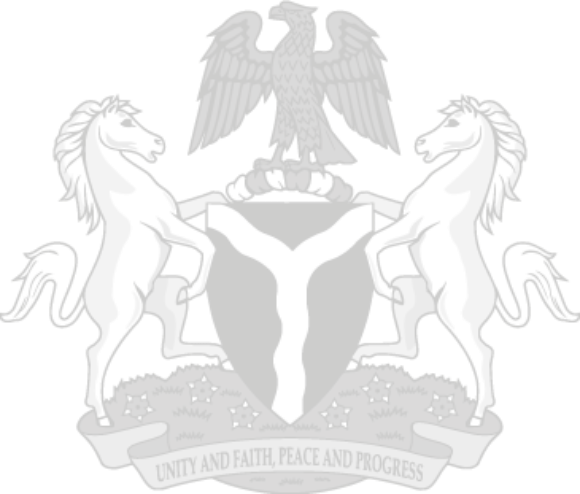## Key Highlights

Nigeria’s Merchandise Trade grew as imports increased significantly and showed a marginal growth in export, resulting in an unfavorable trade balance in Q3, 2021:

Total Trade increased by 10.43% in Q3, 2021 compared to Q2, 2021 and 58.59% year-on-year.

IMPORTS

Total Imports value stood at N8,153.79 billion in Q3 2021 was 17.32% higher than Q2 2021 and 51.47% higher than Q3 2020.

• The value ofImported Agricultural goods was 21.01% higher than the value recorded in Q2, 2021 and 74% higher than Q3 2020.
• The value of Raw material imports was 9.08% more than Q2, 2021, and 29.06% more than the value in Q3 2020.
• Solid Minerals imports in Q3 2021 increased by 10.93% compared to Q2 2021 and 53.42% compared to Q3 2020.
• Energy goods imports in Q3 2021 were 408.51% more than Q2 2021 and 24.14% more than Q3 2020.
• Manufactured goods imports value was 13.98% higher in Q3 2021 than the level in Q2 2021 and 42.73% more than Q3 2020.
• Oil products imports value was 34.60% more than in Q22021 and 113.18% higher than Q3 2020.

EXPORTS

The value of Total Exports stood at N5,130.30billion in Q3, 2021 representing an increase of 1% over Q2 2021 and 71.38% over Q3 2020.

• Agricultural goods export value in Q3, 2021 was 5.9% lower than Q2 2021 but 31.0% higher than Q3 2020.
• Raw material exports value increased by 135.2% in Q3 2021 against the level in Q2 2021 and 1,241.1% higher than Q3 2020.
• Solid Minerals exports value in Q3 2021, increased by 22.7% compared to Q2 2021 and 222.8% compared to Q3 2020.
• Energy goods exports value in Q3 2021 was 58% lower than Q2 2021 and 34.5% lower than the value in Q3 2020.
• Manufactured goods exports were 39.4% more than the value in Q2 2021 and 121.6% higher than Q3 2020.
• Crude Oil exports in Q3 2021 were 1.3% lower than the value recorded in Q2 2021 but 66% higher than Q3 2020.
• Other oil products exports in Q3 2021 were 3.6% more in value than in Q2 2021 and 57.8% higher than Q3 2020.

Data source: National Bureau of Statistics

### INFOGRAPHICS### Portal & Apps